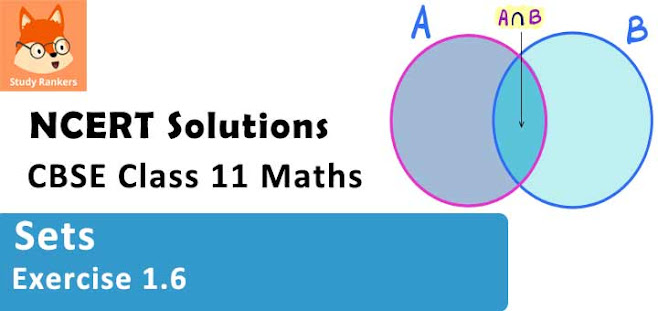# Class 11 Maths NCERT Solutions for Chapter 1 Sets Exercise 1.6### Sets Exercise 1.6 Solutions

1. If X and Y are two sets such that n(X) = 17, n(Y) = 23 and n(X ∪ Y) = 38, find n(X ∩Y).

Solution

It is given that:
n(X) = 17, n(Y) = 23, n(X ∪ Y) = 38
n(X ∩ Y) = ?
We know that:
n(X ∪ Y)= n(X) + n(Y) - n(X ∩ Y)
∴ 38 = 17 + 23 - n(X ∩ Y )
⇒ n (X ∩ Y) = 40 - 38 = 2
∴ n(X ∩ Y) = 2

2. If X and Y are two sets such that X ∪Y has 18 elements, X has 8 elements and Y has 15 elements; how many elements does X ∩Y have?

Solution

It is given that :
n(X ∪ Y) = 18, n(X) = 8, n(Y) = 15
n(X ∩ Y) = ?
We know that :
n(X ∪ Y)= n(X) + n(Y) - n(X ∩ Y)
∴ 18 = 8 + 15 - n(X ∩ Y)
⇒ n(X ∩ Y) = 23 - 18 = 5
∴ n(X ∩ Y) = 5

3. In a group of 400 people, 250 can speak Hindi and 200 can speak English. How many people can speak both Hindi and English?

Solution

Let H be the set of people who speak Hindi, and
E be the set of people who speak English
∴ n(H ∪ E) = 400, n(H) = 250, n(E) = 200
n(H ∩ E) = ?
We know that:
n(H ∪ E) = n(H) + n(E) – n(H ∩ E)
∴ 400 = 250 + 200 – n(H ∩ E)
⇒ 400 = 450 – n(H ∩ E)
⇒ n(H ∩ E) = 450 – 400
∴ n(H ∩ E) = 50
Thus, 50 people can speak both Hindi and English.

4. If S and T are two sets such that S has 21 elements, T has 32 elements, and
S ∩ T has 11 elements, how many elements does S ∪ T have?

Solution

It is given that:
n(S) = 21, n(T) = 32, n(S ∩ T) = 11
We know that:
n (S ∪ T) = n (S) + n (T) – n (S ∩ T)
∴ n (S ∪ T) = 21 + 32 – 11 = 42
Thus, the set (S ∪ T) has 42 elements.

5. If X and Y are two sets such that X has 40 elements, X ∪Y has 60 elements and X ∩Y has 10 elements, how many elements does Y have?

Solution

It is given that:
n(X) = 40, n(X ∪ Y) = 60, n(X ∩ Y) = 10
We know that:
n(X ∪ Y) = n(X) + n(Y) – n(X ∩ Y)
∴ 60 = 40 + n(Y) – 10
∴ n(Y) = 60 – (40 – 10) = 30
Thus, the set Y has 30 elements.

6. In a group of 70 people, 37 like coffee, 52 like tea, and each person likes at least one of the two drinks. How many people like both coffee and tea?

Solution

Let C denote the set of people who like coffee, and
T denote the set of people who like tea
n(C ∪ T) = 70, n(C) = 37, n(T) = 52
We know that:
n(C ∪ T) = n(C) + n(T) – n(C ∩ T)
∴ 70 = 37 + 52 – n(C ∩ T)
⇒ 70 = 89 – n(C ∩ T)
⇒ n(C ∩ T) = 89 – 70 = 19
Thus, 19 people like both coffee and tea.

7. In a group of 65 people, 40 like cricket, 10 like both cricket and tennis. How many like tennis only and not cricket? How many like tennis?

Solution

Let C denote the set of people who like cricket, and
T denote the set of people who like tennis
∴ n(C ∪ T) = 65, n(C) = 40, n(C ∩ T) = 10
We know that:
n(C ∪ T) = n(C) + n(T) – n(C ∩ T)
∴ 65 = 40 + n(T) – 10
⇒ 65 = 30 + n(T)
⇒ n(T) = 65 – 30 = 35
Therefore, 35 people like tennis.
Now,
(T – C) ∪ (T ∩ C) = T
Also,
(T – C) ∩ (T ∩ C) = Φ
∴ n (T) = n (T – C) + n (T ∩ C)
⇒ 35 = n (T – C) + 10
⇒ n (T – C) = 35 – 10 = 25
Thus, 25 people like only tennis.

8. In a committee, 50 people speak French, 20 speak Spanish and 10 speak both Spanish and French. How many speak at least one of these two languages?

Solution

Let F be the set of people in the committee who speak French, and
S be the set of people in the committee who speak Spanish
∴ n(F) = 50, n(S) = 20, n(S ∩ F) = 10
We know that:
n(S ∪ F) = n(S) + n(F) – n(S ∩ F)
= 20 + 50 – 10
= 70 – 10 = 60
Thus, 60 people in the committee speak at least one of the two languages.